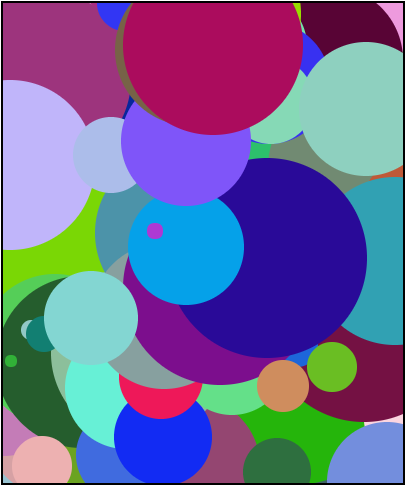## Using Timers

Timers are a key part of creating animations. As such, they are often used to create fun and interesting graphical programs. Let's take a look at one such program, called "Random Circles."

``````/**
* The Random Circles Program
*/

var MAX_CIRCLES = 1000;
var counter = 0;

function start(){
setTimer(draw, 50);
}

function draw(){
Randomizer.nextColor(),
Randomizer.nextInt(0, getWidth()),
Randomizer.nextInt(0, getHeight()));

counter++;

if(counter == MAX_CIRCLES){
stopTimer(draw);
}
}

circle.setColor(color);
circle.setPosition(x, y);
}
``````Let's start by examining the timer. The timer is created at the very beginning of the program in the `start` function.

## <!--

What function is the timer calling?

• ( ) `makeBall`
• (x) `draw`
• ( ) `drawCircle`
• ( ) `start`
• ( ) `timer`

There is no `makeBall` function in the code. Correct! The `drawCircle` function is called by the `draw` function, not the timer. The `start` function is not called by the timer. The timer cannot call itself.

How often does the timer call this function?

• ( ) Every minute
• ( ) Every 50 seconds
• ( ) Every 5 milliseconds
• ( ) Every 5 seconds
• (x) Every 50 milliseconds

Incorrect. The delay is given in milliseconds, not seconds. Note that it is 50 and not 5.

Correct!

-->

## Stopping a Timer

Timers will continue to run until they are given the command to stop. A timer can be stopped by issuing the `stopTimer(functionName)` command. The `functionName` should be the name of the function that was originally passed to the timer when creating it with `startTimer()`.

For example, Karel could create a timer that called a `giveTreat` function every 1000 milliseconds:

``````startTimer(giveTreat, 1000);
``````

Karel could then stop the timer by issuing the following command:

``````stopTimer(giveTreat);
``````

## <!--

In the Random Circles program above, what command will stop the timer from drawing new circles?

• ( ) `stopTime(draw)`
• ( ) `stop(draw)`
• (x) `stopTimer(draw)`
• ( ) `stopTimer(draw, 50)`
• ( ) `stopTimer(drawCircle)`

Close, but the command is `stopTimer`, not `stopTime`. The proper command is `stopTimer`. Correct! You should only pass the name of the function being called, not a delay. You'll need to give the proper function name that the timer is calling.

When does the timer stop in the Random Circles program?

• (x) When the `counter` reaches 1000.
• ( ) When the screen is filled with circles.
• ( ) It does not stop until there are 990 circles.
• ( ) The timer stops when there are no more circles.
• ( ) We can't stop, we won't stop.

Correct! Notice the `counter` variable is checked each time `draw` is called. The value of `MAX_CIRCLES` is 1000, not 990. There is not a built in number of circles that can be drawn. We run things, things don't run we.

-->

## Using Multiple Functions with a Timer

Programs often need to call multiple functions every tick of the timer. However, the call to `startTimer` can only take one single function. This code would not work for Karel:

``````startTimer(giveTreat, giveToy, takeKarelForWalk, 1000);
``````

Instead of passing multiple functions when calling `startTimer`, Karel will need to create one function that then makes calls to the other functions:

``````startTimer(makeKarelHappy, 1000);

...

function makeKarelHappy(){
giveTreat();
giveToy();
takeKarelForWalk();
}
``````

With this code, the `makeKarelHappy` function will be called every 1000 milliseconds. That function then calls each of Karel's other functions. Notice that in the Random Circles program, the timer calls the `draw` function, which then calls `drawCircle`.